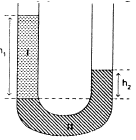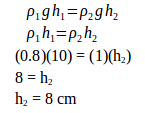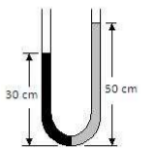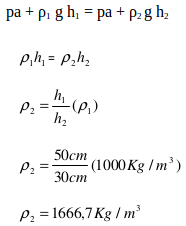# Manometer tube – problems and solutions

1. A manometer tube is filled with two type of liquids. The density of liquid 1 is ρ1 = 0.8 g.cm-3, and the density of liquid 2 is ρ2 = 1 g.cm-3, and height h1 = 10 cm, then what is the height of h2.

Known :Density of liquid 1 = 0.8 g.cm-3

Density of liquid 2 = 1 g.cm-3

Height 1 = 10 cm

Wanted: Height 2

Solution :[irp]

2. The height of the water is 50 cm, and the height of another liquid is 30 cm. What is the density of another liquid?

Known :

Acceleration due to gravity (g) = 10 m/s2The height of water (h1) = 50 cm

Density of water 1) = 1.00 x 103 kg/m3 = 1000 kg/m3

Height of another liquid (h2) = 30 cm

Wanted : The density of another liquid.

Solution :

pa + ρ1 g h1 = pa + ρ2 g h2